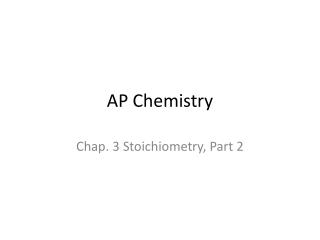DownloadDownload PresentationAP Chemistry

# AP Chemistry

Télécharger la présentation## AP Chemistry

- - - - - - - - - - - - - - - - - - - - - - - - - - - E N D - - - - - - - - - - - - - - - - - - - - - - - - - - -
##### Presentation Transcript

1. AP Chemistry Chap. 3 Stoichiometry, Part 2

2. 3.6 Chemical Equations (p. 100)- shows a chemical change. Reactants on the LHS, products on the RHS. Bonds have been broken, and new bonds have been formed. Law of Conservation of Matter applies (must have same number and type of atoms on the product side and on the reactant side). Use symbols to indicate state of matter: • (s) solid • (l) liquid • (g) gas • (aq) dissolved in water (aqueous solution)

3. Problem: Write the following equations: Hydrogen and nitrogen form ammonia N2+ H2 NH3 Hydrogen and oxygen form water H2+ O2 H2O Magnesium and oxygen form magnesium oxide Mg + O2MgO Hydrochloric acid and sodium hydroxide react to form sodium chloride and water HCl+ NaOHNaCl + H2O

4. 3.7 Balancing Chemical Equations (p. 102) (Balance the above equations) N2 + 3H2 2NH3 2H2 + O2 2H2O 2Mg + O2 2MgO HCl + NaOHNaCl + H2O (already balanced)

5. 3.8 Stoichiometric Calculations: Amounts of Reactants & Products (p. 107) Because of the necessity of balancing chemical equation, we realize that chemical reactions occur according to proportion or by mole ratio. Mass moles or moles  mass may complicate the calculation, but the principle is consistent. Problem: For the reaction: CH4(g) + 2O2(g)  CO2(g) + 2H2O(g) How many moles of carbon dioxide are formed when 40. moles of oxygen (O2) is consumed? 40. mol O2 x (1 mol CO2/2 mol O2) = 20. Mol CO2

6. How many moles of methane are needed to form 200. moles of water? 200. mol H2O x (1 mol methane/2 mol H2O) = 100. Mol CH4 How many moles of oxygen (O2) combine with 0.050 mole of methane? 0.050 mol CH4 x (2 mol O2/ 1 mol CH4) = 0.10 mol O2

7. 3.9 Calculations Involving a Limiting Reactant (p. 111) Because chemical must react in stoichiometric quantities and because sometimes your reactants are not in stoichiometric quantities, there will be a limiting reactant (the reactant which is consumed completely before the other(s). Once that happens, the reaction stops. (Law of Conservation of Matter). The other reactant(s) are in excess. The amount of product produced is directly determined by the limiting reactant.

8. Problem: How many grams of water are produced when 10.0 grams of H2 is mixed with 100.0 grams of O2 and the mixture is ignited with a spark? (** need a balanced equation: 2H2 + O2 2 H2O 10.0 g H2 x (1 mol H2/2 g H2) x (1 mol O2/2 mol H2) x (32 g O2/1 mol O2) = 80.0 g O2 needed. (have 100.0 O2, therefore, H2 is Limiting Reactant) 10.0 g H2 x (1 mol H2/2 g H2) x (2 mol H2O/2 mol H2) x (18 g H2O/1 mol H2O) = 90.0 g H2O produced

9. Theoretical & percent Yield (p. 118)- comparison of the amount actually produced in a chemical reaction with the calculated (or theoretical) amount. (NOTE: not the same as the % error formula!!) % yield = (actual yield/ theoretical yield) x 100% % yield should always be less than 100%. (actual yield should be less than theoretical yield). Problem: in the above problem (not the embedded problem w/ phosphine, but the problem above that), what is the % yield if only 80.0 grams of H2O is produced? % yield = (80.0 g/ your answer) x 100% = _____________________